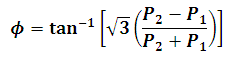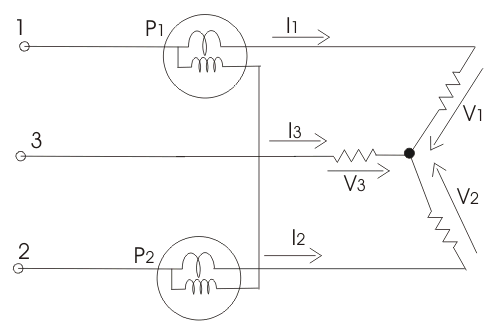# Power Factor Using Two Watt-meter Calculator

This Calctown Calculator calculates the power factor of a three phase circuit using the two watt-meter  method.

kW
kW

#### Result

deg